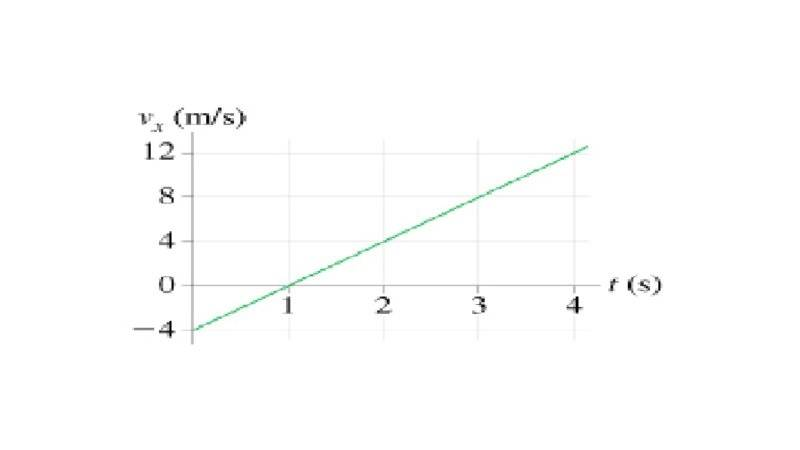# Position from velocity time graph## Homework Statement

A particle starts from x_0 = 14m at t_0 = 0 and moves with the velocity graph shown
What is the object's position at t = 2 s?

## Homework Equations

Area under velocity time graph is the displacement of the particle.

## The Attempt at a Solution

The part that confuses me is where the velocity goes negative. I think that I should assign negative value to the portion where v_x is negative.
So from t=1 to t=2: (1/2)(1*4) = 2m
from t=0 to t=1 it is negative: - (1/2)(1*4) = -2m
-2m+2m = 0m = no displacement?
Is this correct before I try using calculus to solve same problem? Thanks!

sorry I forgot the initial velocity = 14m/s.
So objects position after 2s is 0m + 14m = 14m

#### Attachments

•Homework Helper
Gold Member
2020 Award
Correct, with one minor correction: Second to last line: Object's initial position is ## x_o=14 ## m. The initial velocity is not 14 m/sec.

Thanks for the help. So for the calculus version Im again confused about what to do with the negative part.
First the equation for the velocity is v_x = 4t (because slope is 4/1 = 4)
So if I intergrate that I get x = 2t^2 + c
From x=1 to x= 2 is 1s: x = 2(1)^2 = 2m (Im not sure what the c represents)
I know that from x=0 to x=1 also = 1s, and that it is the same equation, but how do I represent the negative so that it will cancel, as like the algebra version? thanks

Homework Helper
Gold Member
2020 Award
These are definite integrals. See (3 lines) below. ## \\ ## The correct expression for ## v_x ## is ## v_x(t)=-4+4t ##.## \\ ## (You can write ## \frac{y-y_1}{x-x_1}=\frac{y_2-y_1}{x_2-x_1} ##, where you use any two points ## (x_1,y_1) ## and ## (x_2,y_2) ##. In this case ## y ## is ## v ##, and ## x ## is ## t ##). ## \\ ## The distance ## \Delta x ## it goes is ## \Delta x=\int\limits_{0}^{2} v_x(t) \, dt ##, (as previously mentioned, it's a definite integral, i.e. one that has its limits posted in the expression, and thereby there is no constant of integration to add to the result like there is with an indefinite integral). ## \\ ## Finally, ## x_{final}=x_o +\Delta x ##. ## \\ ## I'll let you perform the integral. ## \\ ## You could also write the integral as ## \Delta x=\int\limits_{0}^{1} v_x(t) \, dt +\int\limits_{1}^{2} v_x(t) \, dt ## if you want to sum these two parts separately.

Last edited:
Ray Vickson
Homework Helper
Dearly Missed
Thanks for the help. So for the calculus version Im again confused about what to do with the negative part.
First the equation for the velocity is v_x = 4t (because slope is 4/1 = 4)
So if I intergrate that I get x = 2t^2 + c
From x=1 to x= 2 is 1s: x = 2(1)^2 = 2m (Im not sure what the c represents)
I know that from x=0 to x=1 also = 1s, and that it is the same equation, but how do I represent the negative so that it will cancel, as like the algebra version? thanks

If positive velocities/displacements are to the right and negative ones to the left, your velocity graph has the particle moving to the left from time 0 to 1. The distance it moves left in that time is the magnitude of the area between the v-graph and the t-axis. (Note that the distance moved is positive and the magnitude of the area is also positive---however, the displacement is negative!) At t=1 the particle starts moving to the right, and between t=1 and t=2 the distance it moves right equals the area under the v-graph and the t-axis. So, between t = 1 and t = 2 the particle just moves back to its starting position.